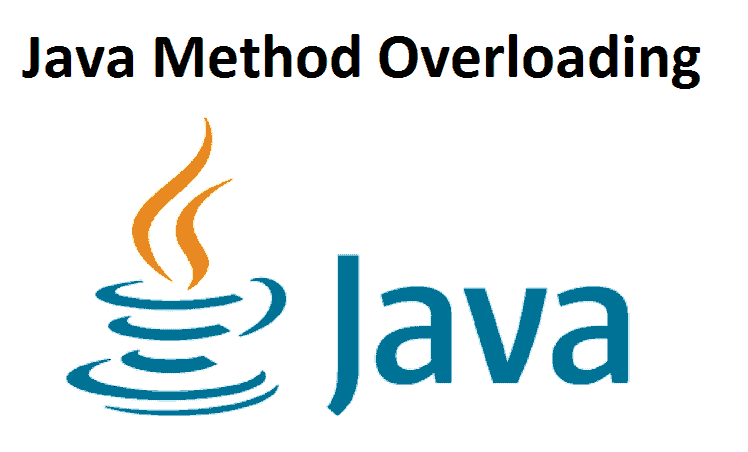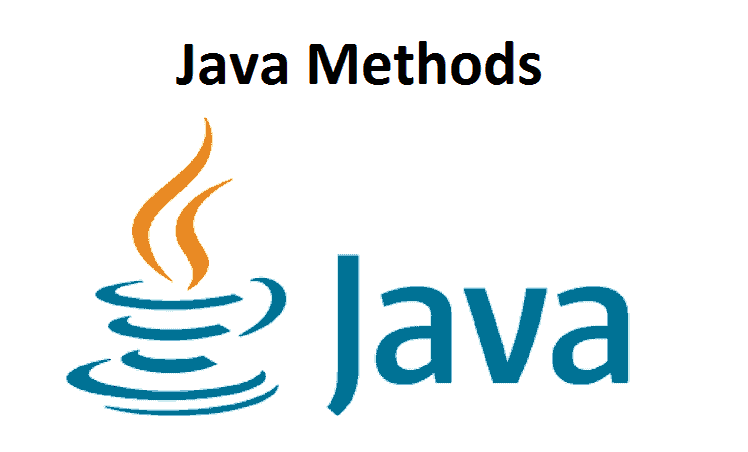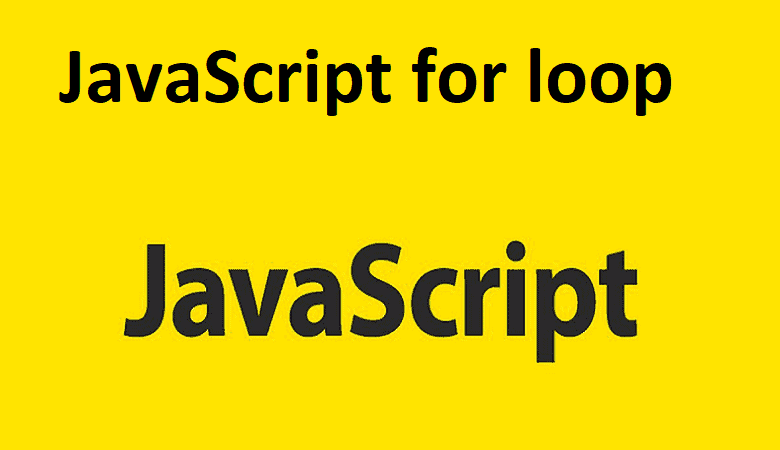#### JavaScript for loop

In this tutorial, you will learn about loops and for loops in JavaScript with the help of examples. Loops are ...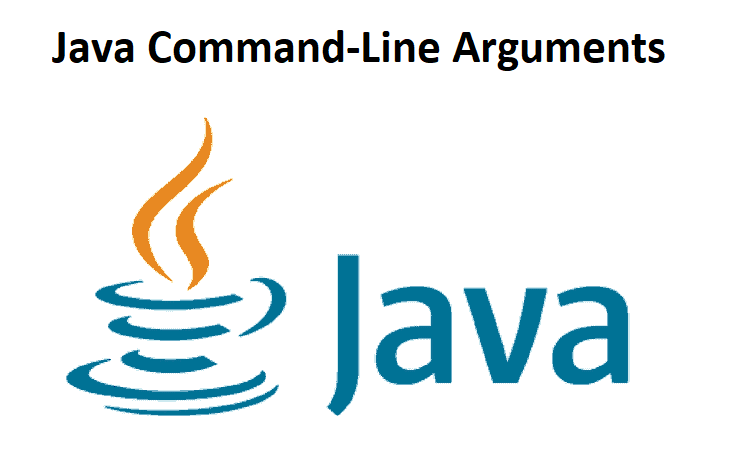#### Java Command-Line Arguments

In this tutorial, we will learn about the Java command-line arguments with the help of examples. The command-line arguments in ...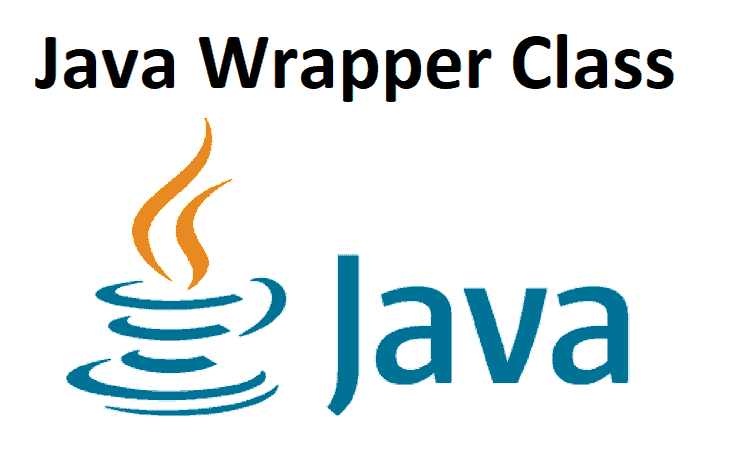#### Java Wrapper Class

In this tutorial, we will learn about the Java Wrapper class with the help of examples. The wrapper classes in ...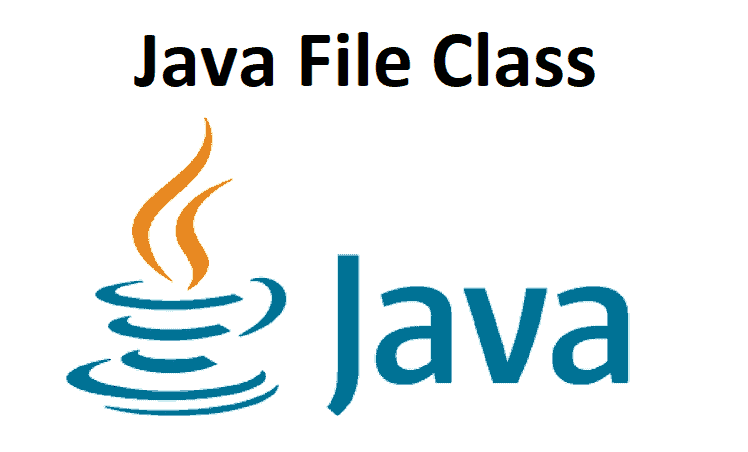#### Java File Class

In this tutorial, we will learn about Java file and their various activities with the help of examples. The File ...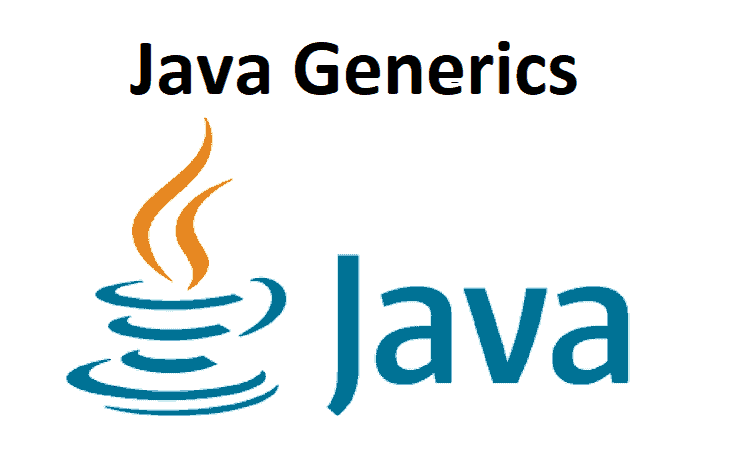#### Java Generics

In this tutorial, we will learn about Java Generics, how to make generics class and techniques, and its advantages with ...#### Java Lambda Expressions

Java Lambda Expressions In this article, we will learn about Java lambda expression and the use of lambda expression with ...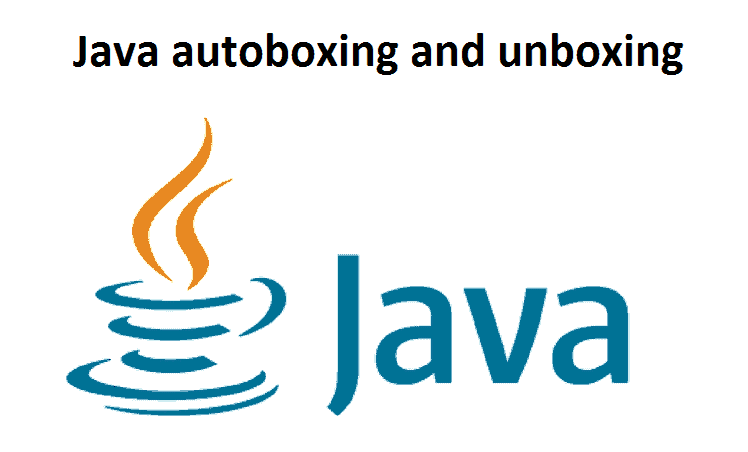#### Java autoboxing and unboxing

In this tutorial, we will learn about Java autoboxing and unboxing with the help of examples. Java Autoboxing - Primitive ...#### Java Type Casting

In this tutorial, we will find out about Java Type Casting and its sorts with the help of examples. Before ...#### Java Scanner Class

In this tutorial, we will learn about the Java Scanner and its strategies with the help of examples. The Scanner ...#### Java PrintWriter Class

In this tutorial, we will learn about Java PrintWriter and its print() and printf() techniques with the help of examples ...#### Java StringWriter Class

In this tutorial, we will learn about Java StringWriter and its subclasses with the help of examples. The StringWriter class ...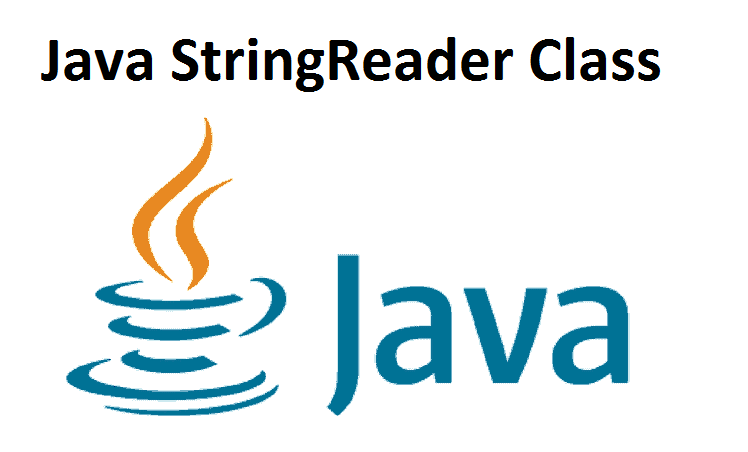In this tutorial, we will learn about Java StringReader and its techniques with the help of examples. The StringReader class ...#### Java BufferedWriter Class

In this tutorial, we will learn about Java BufferedWriter and its strategies with the help of examples. The BufferedWriter class ...In this tutorial, we will learn about Java BufferedReader and its strategies with the help of examples. The BufferedReader class ...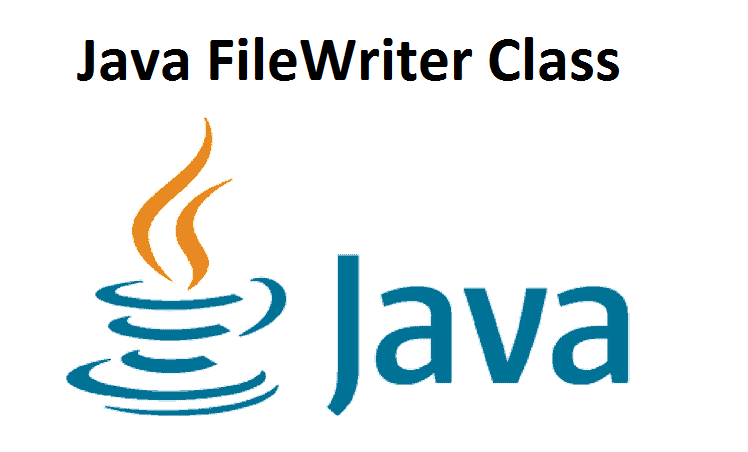#### Java FileWriter Class

In this tutorial, we will learn about Java FileWriter and its strategies with the help of examples. The FileWriter class ...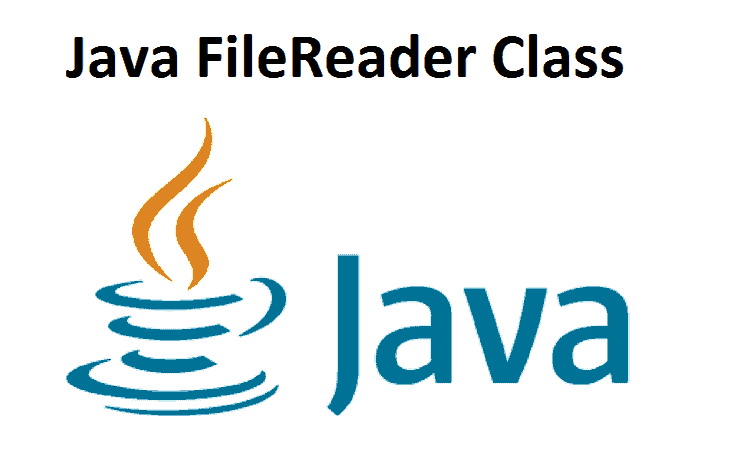In this tutorial, we will learn about Java FileReader and its techniques with the help of examples. The FileReader class ...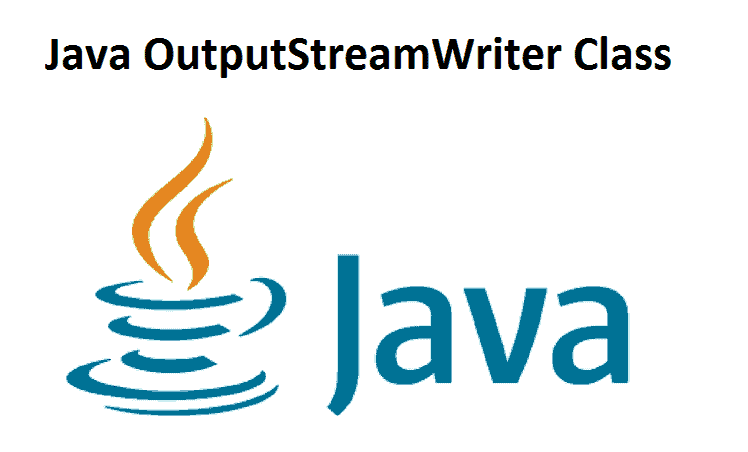#### Java OutputStreamWriter Class

In this tutorial, we will learn about Java OutputStreamWriter and its techniques with the help of examples. The OutputStreamWriter class ...In this tutorial, we will learn about Java InputStreamReader and its strategies with the help of examples. The InputStreamReader class ...#### Java Writer Class

In this tutorial, we will learn about Java Writer, its subclasses, and its strategies with the help of an example ...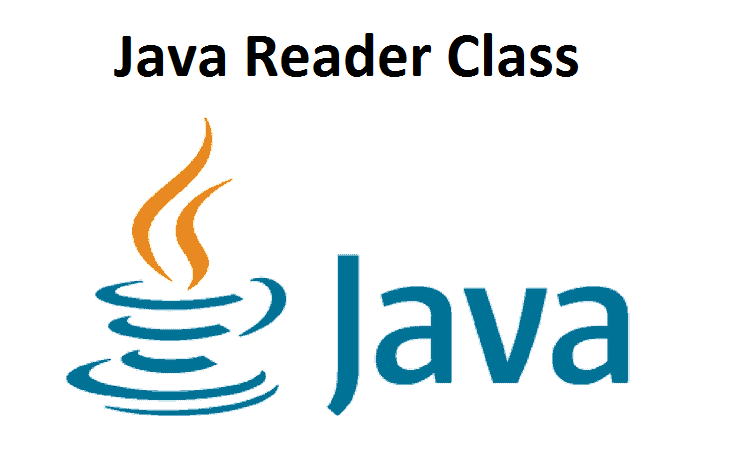In this tutorial, we will learn about Java Reader, its subclasses, and its strategies with the help of an example ...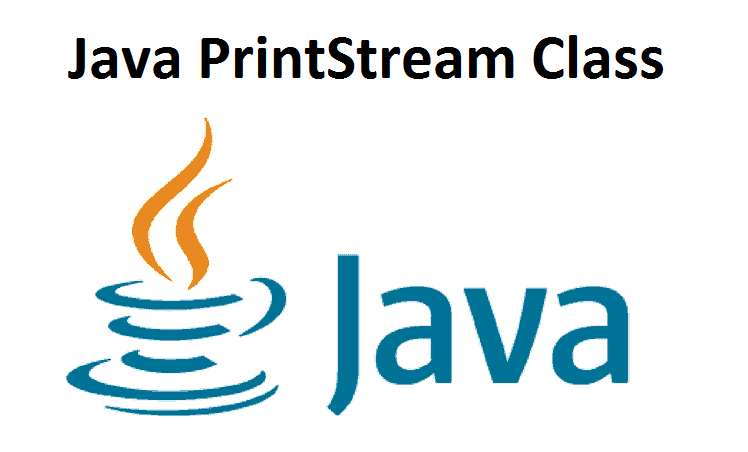#### Java PrintStream Class

In this tutorial, we will learn about the Java PrintStream class and its print() and printf() strategies with the help ...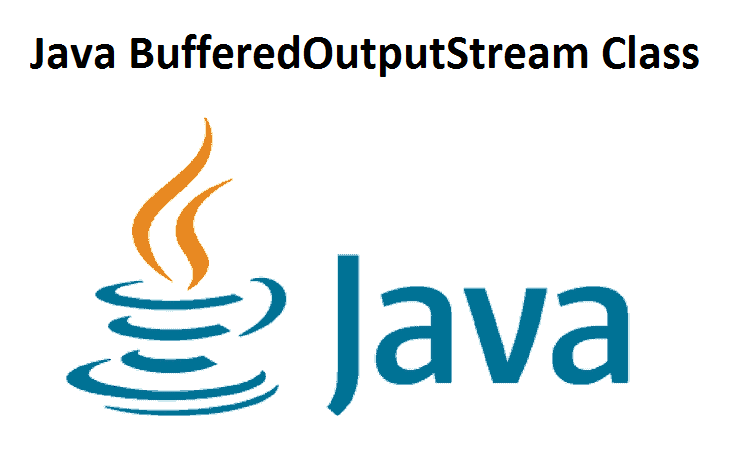#### Java BufferedOutputStream Class

In this tutorial, we will learn about Java BufferedOutputStream and its techniques with the help of examples. The BufferedOutputStream class ...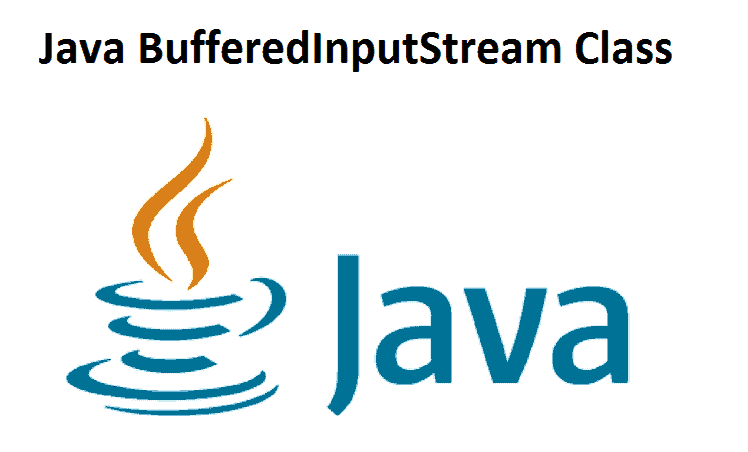#### Java BufferedInputStream Class

In this tutorial, we will learn about Java BufferedInputStream and its strategies with the help of examples. The BufferedInputStream class ...#### Java ObjectOutputStream Class

In this tutorial, we will learn about Java ObjectOutputStream and its strategies with the help of examples. The ObjectOutputStream class ...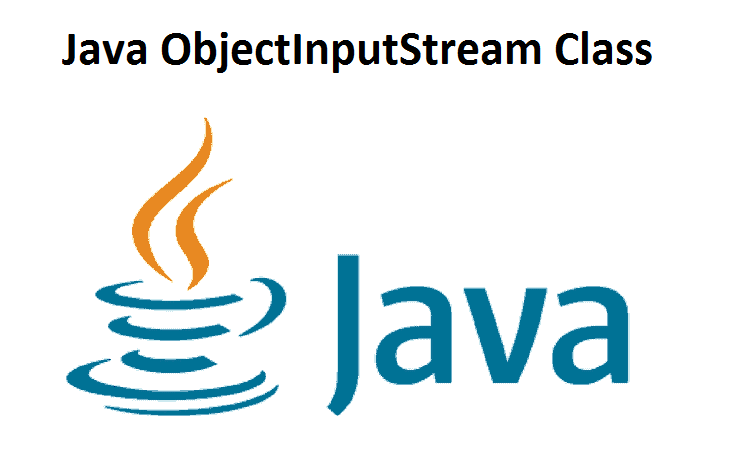#### Java ObjectInputStream Class

In this tutorial, we will learn about Java ObjectInputStream and its strategies with the help of examples. The ObjectInputStream class ...#### Java ByteArrayOutputStream Class

In this tutorial, we will learn about Java ByteArrayOutputStream and its strategies with the help of examples. The ByteArrayOutputStream class ...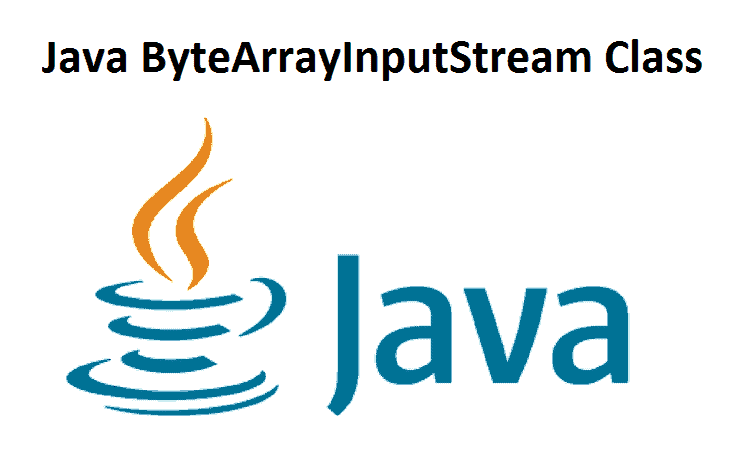#### Java ByteArrayInputStream Class

In this tutorial, we will learn about Java ByteArrayInputStream and its techniques with the help of examples. The ByteArrayInputStream class ...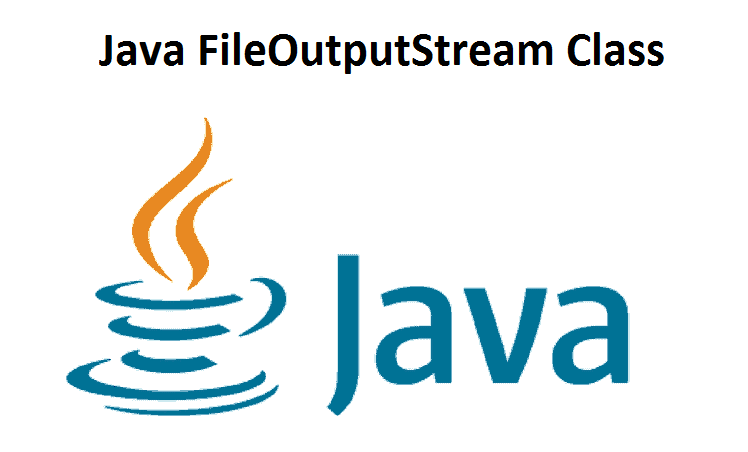#### Java FileOutputStream Class

In this tutorial, we will learn about Java FileOutputStream and its techniques with the help of examples. The FileOutputStream class ...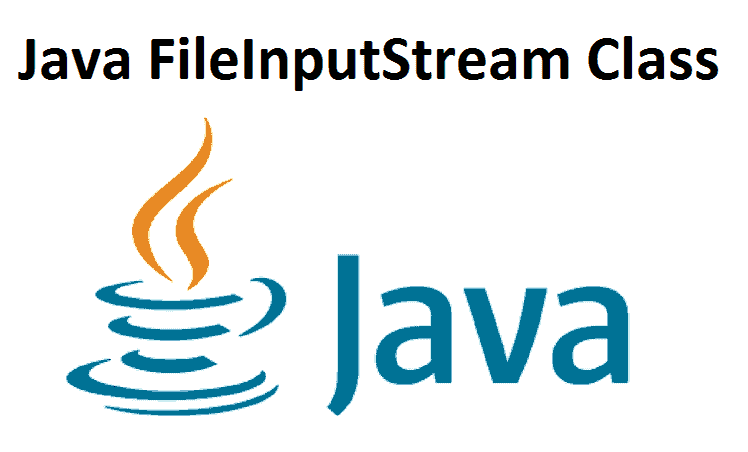#### Java FileInputStream Class

In this tutorial, we will learn about Java FileInputStream and its strategies with the help of examples. The FileInputStream class ...#### Java OutputStream Class

In this tutorial, we will learn about the Java OutputStream and its methods with the help of an example. The ...#### Java InputStream Class

In this tutorial, we will learn about the Java InputStream class and its methods with the help of an example ...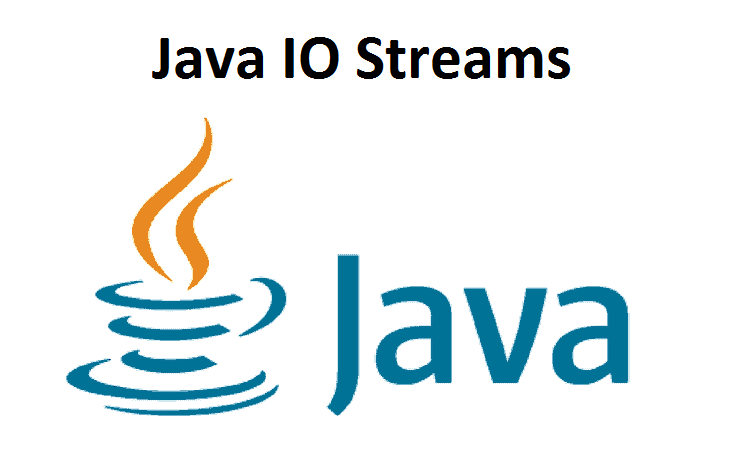#### Java I/O Streams

In this tutorial, we will learn about Java input/output streams and their sorts. In Java, streams are the sequence of ...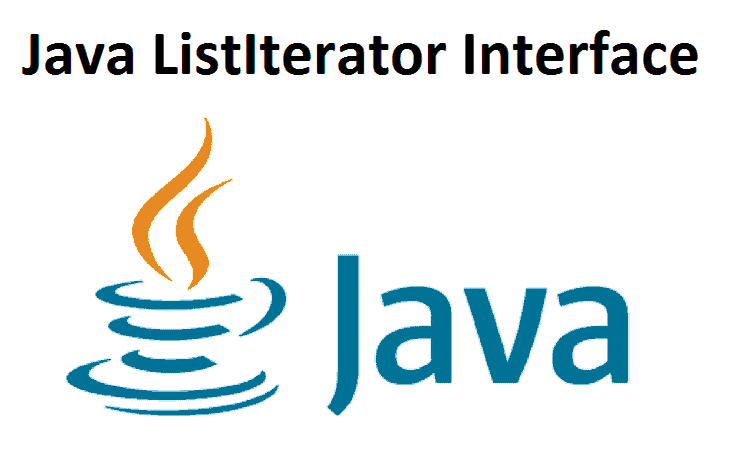#### Java ListIterator Interface

In this tutorial, we will learn about the Java ListIterator interface with the help of an example. The ListIterator interface ...#### Java Iterator Interface

In this tutorial, we will learn about the Java Iterator Interface with the help of an example. The Iterator interface ...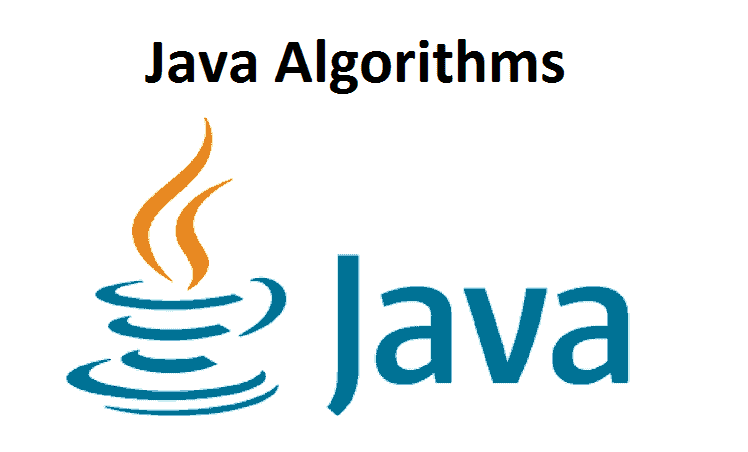#### Java Algorithms

In this tutorial, we will learn about various algorithms furnished by the Java collections framework with the help of examples ...#### Java TreeSet

In this tutorial, we will learn about the Java TreeSet class and its different activities and methods with the help ...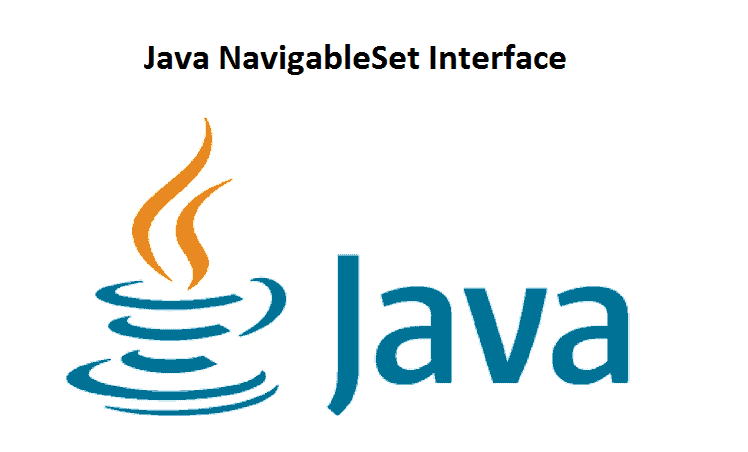#### Java NavigableSet Interface

Java NavigableSet Interface In this tutorial, we will learn about the Java NavigableSet interface and its methods with the help ...#### Java SortedSet Interface

In this tutorial, we will learn about the SortedSet interface in Java and its methods with the help of an ...In this tutorial, we will learn about the Java LinkedHashSet class and its methods with the help of examples. The ...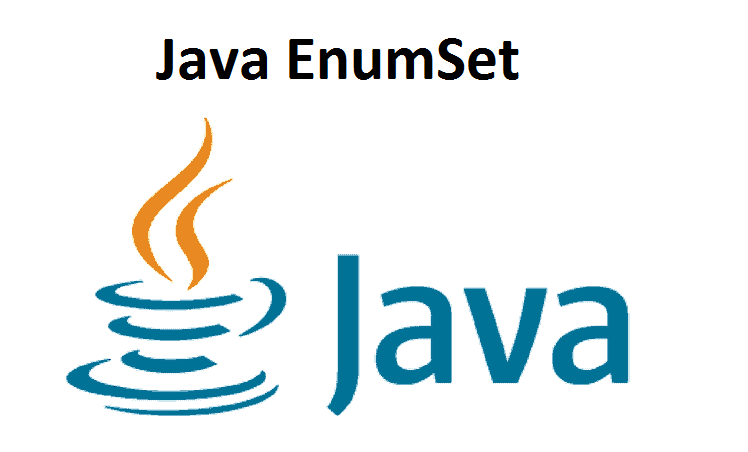#### Java EnumSet

Java EnumSet In this tutorial, we will learn about the Java EnumSet class and its different methods with the help ...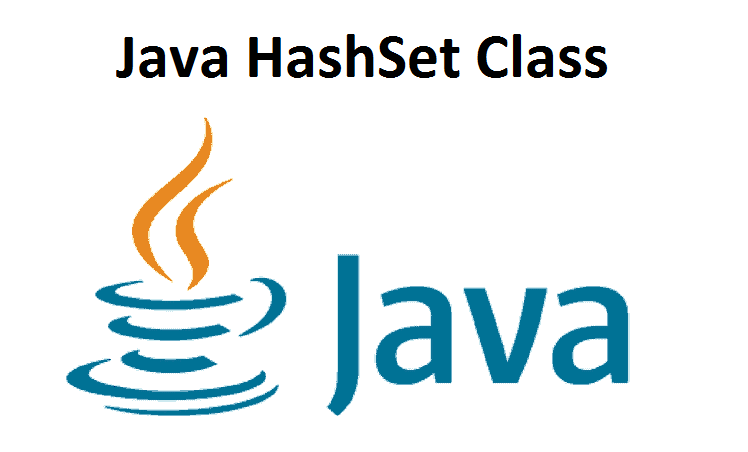#### Java HashSet Class

In this tutorial, we will learn about the Java HashSet class. We will learn about various hash set techniques and ...#### Java Set Interface

Java Set Interface In this tutorial, we will learn about the Set interface in Java and its methods. The Set ...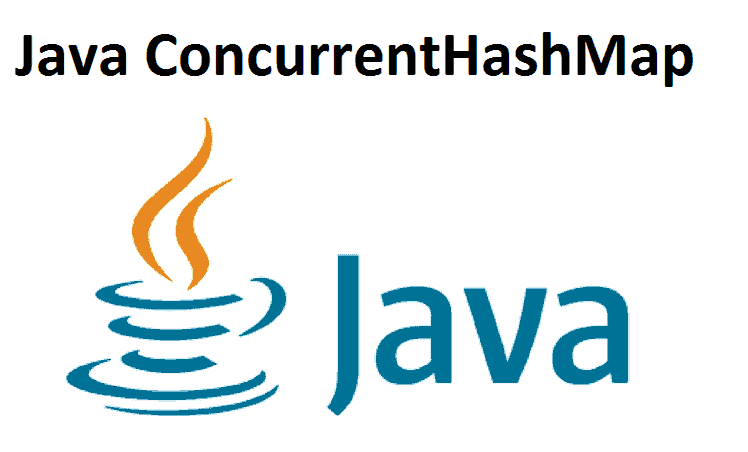#### Java ConcurrentHashMap

Java ConcurrentHashMap In this tutorial, we will learn about the Java ConcurrentHashMap class and its tasks with the help of ...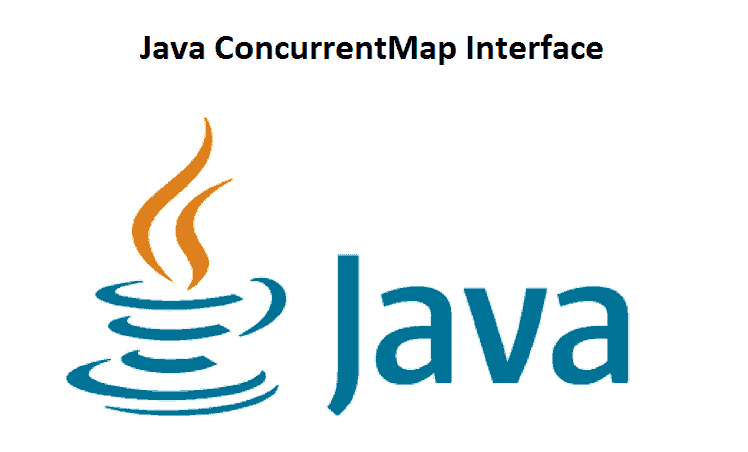#### Java ConcurrentMap Interface

Java ConcurrentMap Interface In this tutorial, we will learn about the Java ConcurrentMap interface and its strategies. The ConcurrentMap interface ...#### Java TreeMap

Java TreeMap In this tutorial, we will learn about the Java TreeMap class and its activities with the help of ...#### Java NavigableMap Interface

Java NavigableMap Interface In this tutorial, we will learn about the Java NavigableMap interface and its techniques with the help ...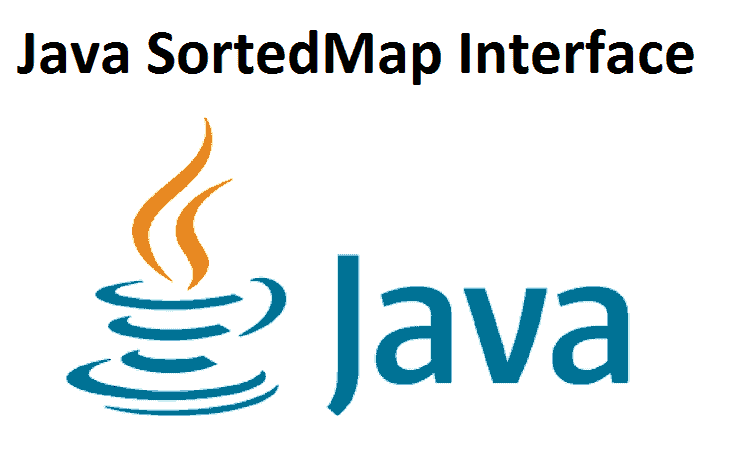#### Java SortedMap Interface

Java SortedMap Interface In this tutorial, we will learn about the Java SortedMap interface and its methods. The SortedMap interface ...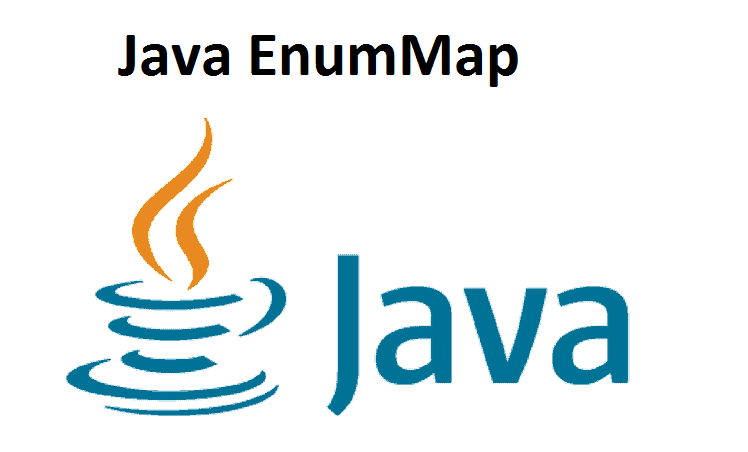#### Java EnumMap

Java EnumMap In this tutorial, we will learn about the Java EnumMap class and its activities with the help of ...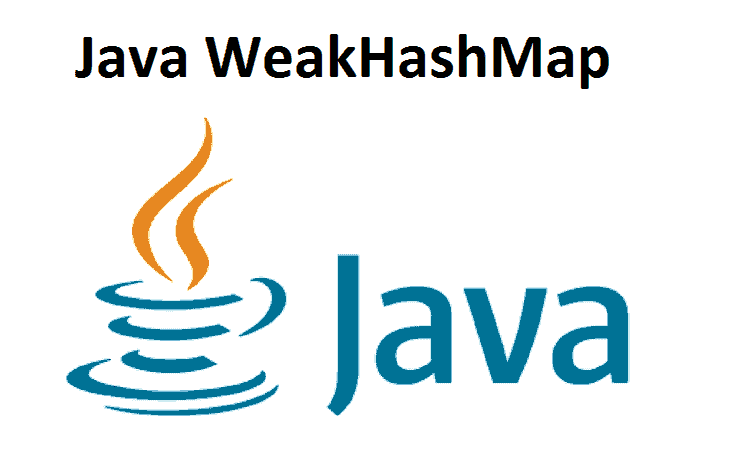#### Java WeakHashMap

Java WeakHashMap In this tutorial, we will learn about Java WeakHashMap and its activities with the help of examples. We ...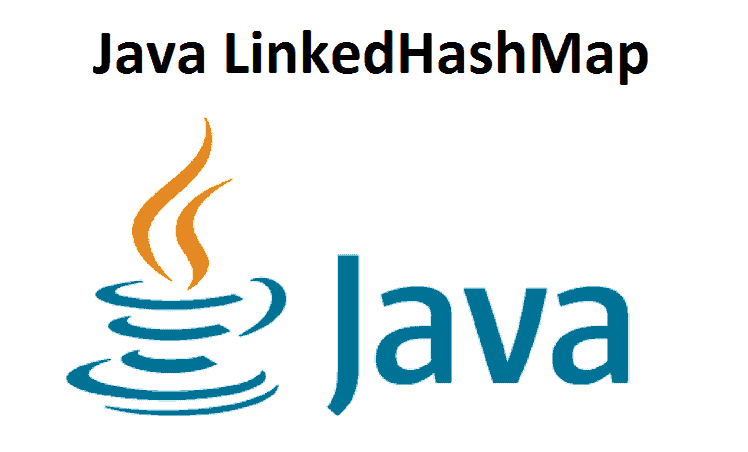Java LinkedHashMap In this tutorial, we will find out about the Java LinkedHashMap class and its activities with the help ...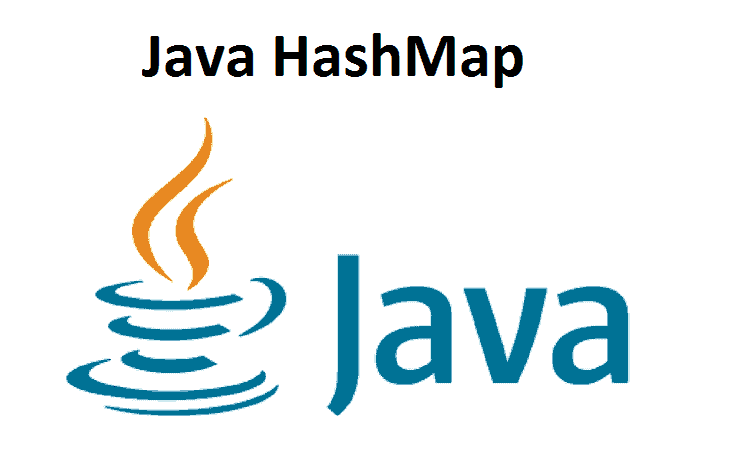#### Java HashMap

Java HashMap In this tutorial, we will learn about the Java HashMap class and its different tasks with the help ...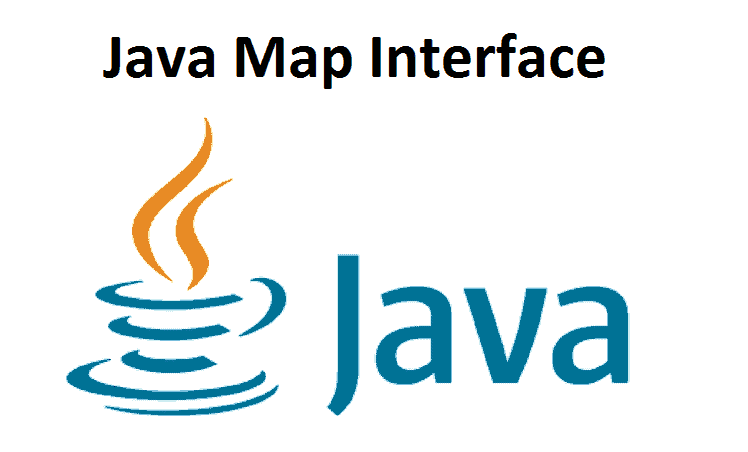#### Java Map Interface

Java Map Interface In this tutorial, we will find out about the Java Map interface and its methods. The Map ...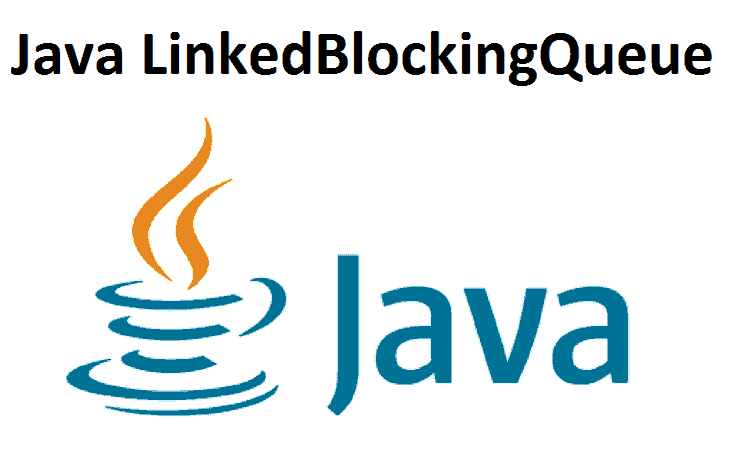Java LinkedBlockingQueue In this tutorial, we will find out about the LinkedBLockingQueue class and its methods with the help of ...#### Java ArrayBlockingQueue

Java ArrayBlockingQueue In this tutorial, we will find out about the ArrayBlockingQueue class, and its methods with the help of ...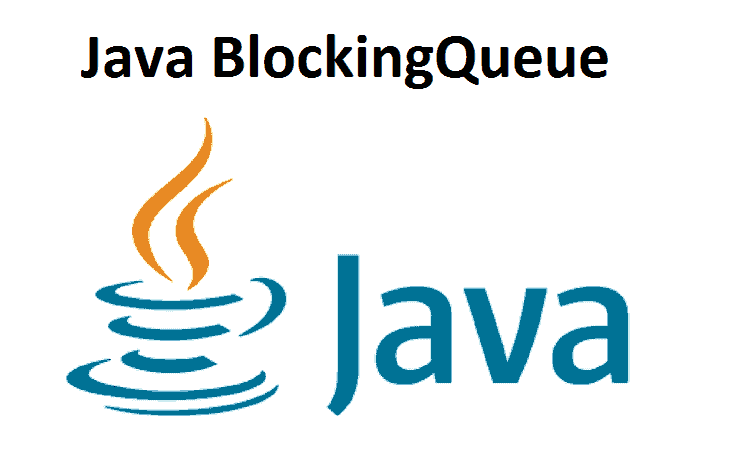#### Java BlockingQueue

Java BlockingQueue In this tutorial, we will find out about the Java BlockingQueue interface and its methods. The BlockingQueue interface ...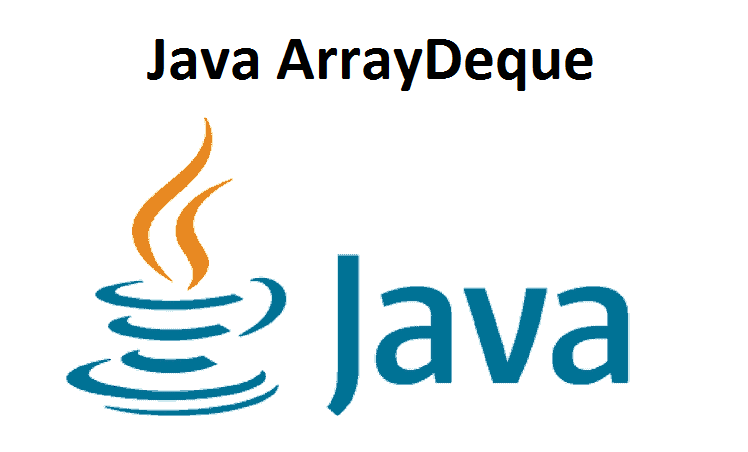#### Java ArrayDeque

Java ArrayDeque Java ArrayDeque: In this tutorial, we will find out about the ArrayDeque class and its methods with the ...In this tutorial, we will find out about the Java LinkedList in detail with the help of examples. The LinkedList ...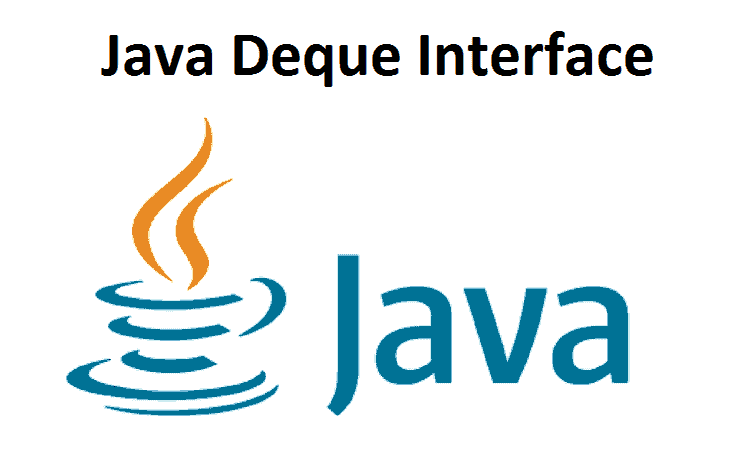#### Java Deque Interface

Java Deque Interface Java Deque Interface: In this tutorial, we will find out about the Deque interface, how to use ...#### Java PriorityQueue

Java PriorityQueue In this tutorial, we will find out about the PriorityQueue class of the Java collections framework with the ...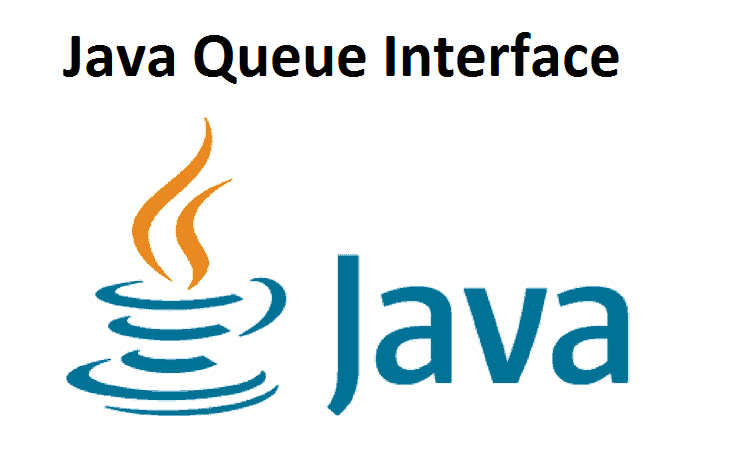#### Java Queue Interface

Java Queue Interface In this tutorial, we will find out about the Java Queue interface and its methods. The Queue ...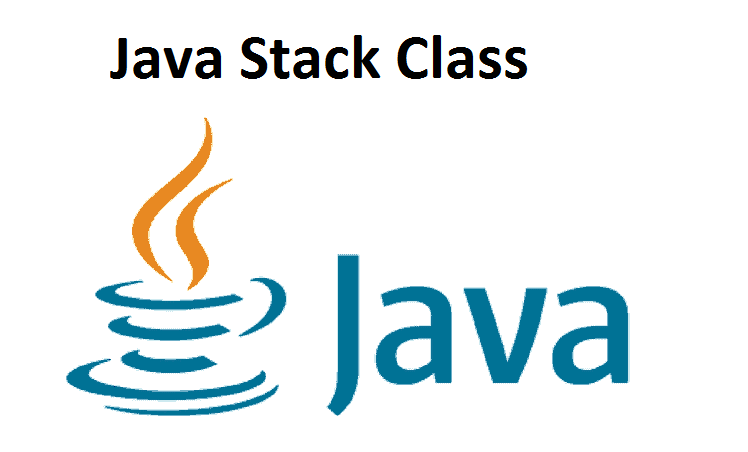#### Java Stack Class

Java Stack Class In this tutorial, we will find out about the Java Stack class and its strategies with the ...#### Java Vector

Java Vector Java Vector: In this tutorial, we will find out about the Vector class and how to use it ...#### Java ArrayList Class

Java ArrayList Class In this tutorial, we will find out about the Java ArrayList class. We will find out about ...#### Java List

Java List In this tutorial, we will find out about the List interface in Java and its methods. In Java, ...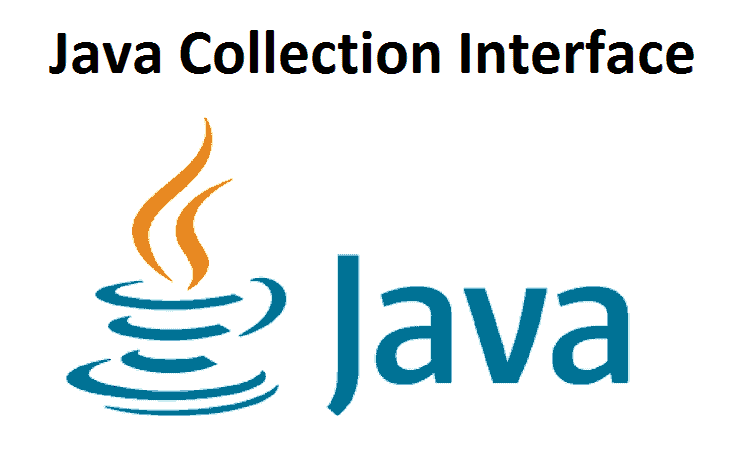#### Java Collection Interface

Java Collection Interface In this tutorial, we will find out about the Java Collection interface and its subinterfaces. The Collection ...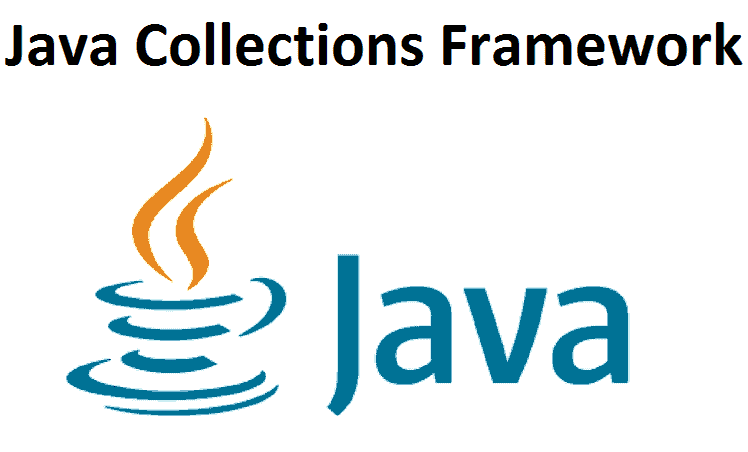#### Java Collections Framework

Java Collections Framework In this tutorial, we will find out about various interfaces of the Java collections framework. The Java ...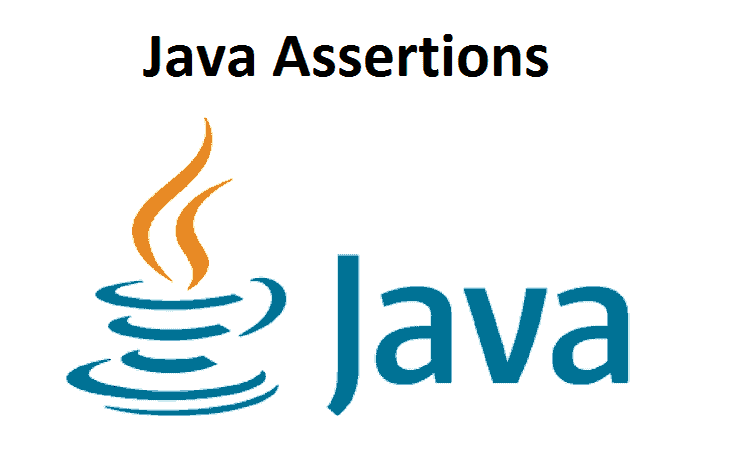#### Java Assertions

Java Assertions Java Assertions: In this tutorial, we will find out about the Java assert statement (Java assertions)) with the ...#### Java Logging

Java Logging In this tutorial, we will find out about Java Logging and its different segments with the help of ...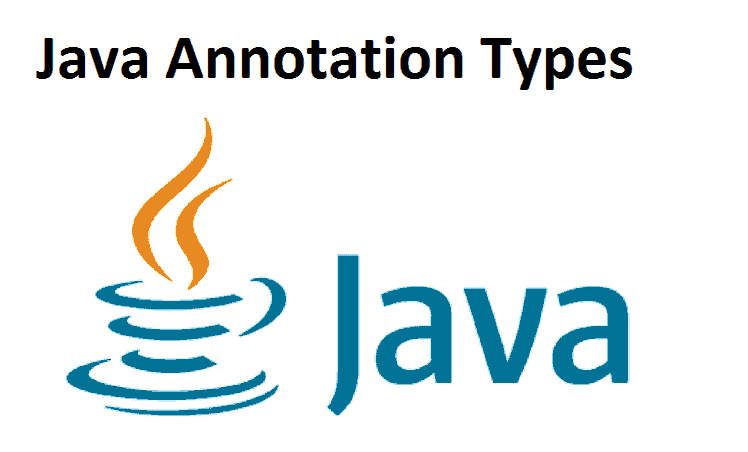#### Java Annotation Types

Java Annotation Types In this tutorial, we will find out about various sorts of Java annotation with the help of ...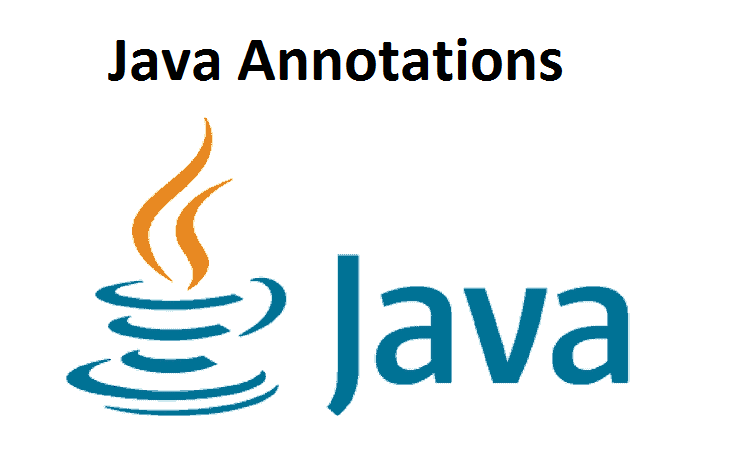#### Java Annotations

Java Annotations In this tutorial, we will realize what annotations are, distinctive Java annotations and how to use them with ...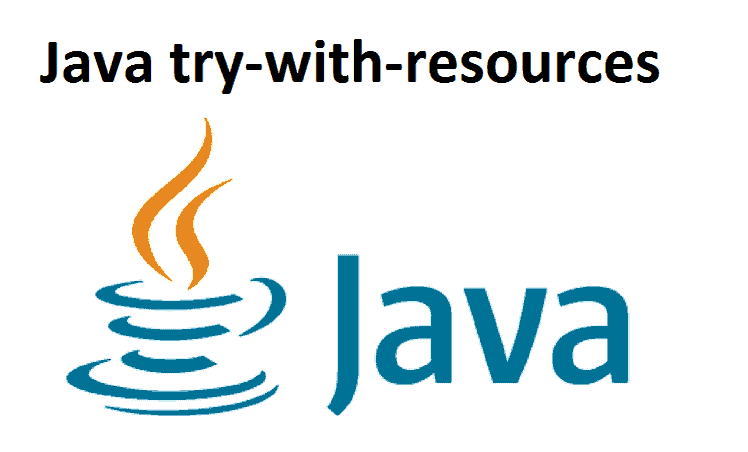#### Java try-with-resources

Java try-with-resources Java try-with-resources: In this tutorial, we will find out about the try-with-resources statement to close resources consequently. The ...#### Java catch Multiple Exceptions

Java catch Multiple Exceptions Java catch Multiple Exceptions: In this tutorial, we will learn to handle numerous exceptions in Java ...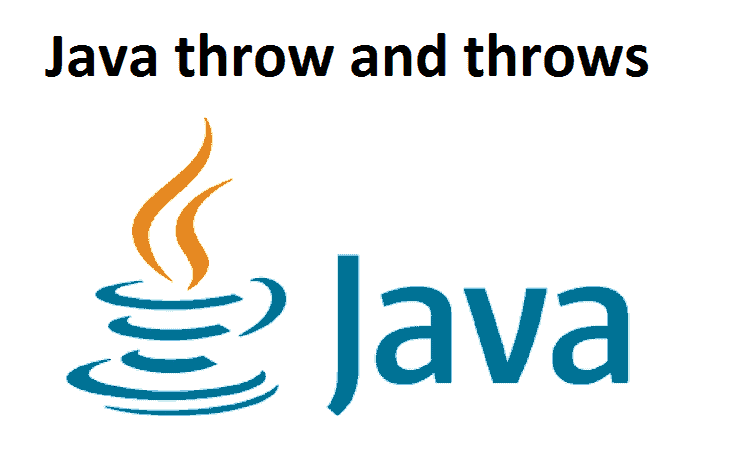#### Java throw and throws

Java throw and throws Java throw and throws: In this tutorial, we will learn to use the throw and throws ...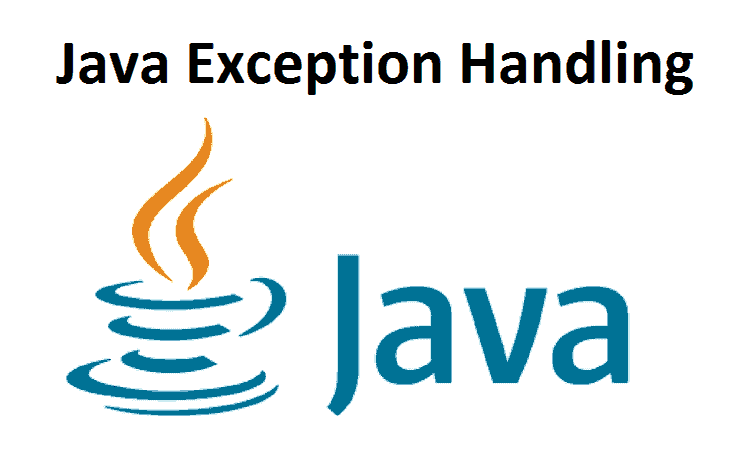#### Java Exception Handling

Java Exception Handling In this tutorial, you will learn to handle exceptions in Java with the help of examples. To ...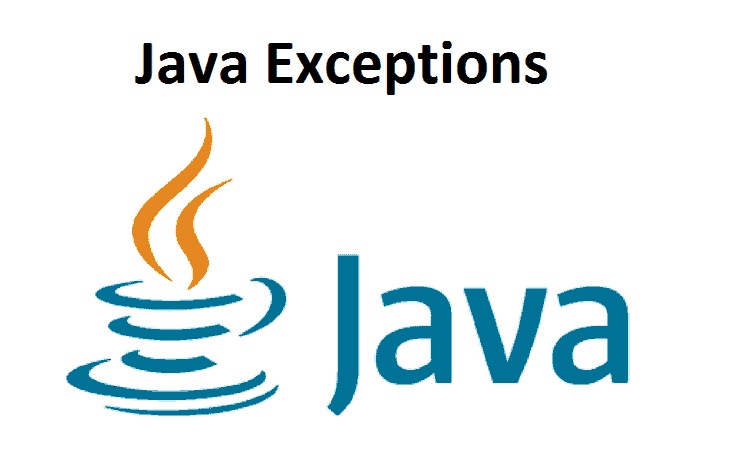#### Java Exceptions

Java Exceptions In this tutorial, we will find out about exemptions in Java. We will cover errors, exemptions, and various ...#### Java Reflection

Java Reflection In this tutorial, we will learn reflection, an element in Java programming that allows us to assess and ...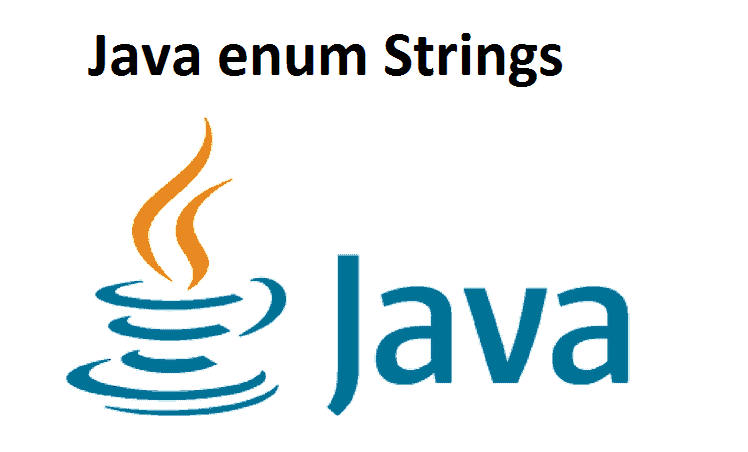#### Java enum Strings

Java enum Strings In this tutorial, we will learn about string values for enum constants. We will likewise learn to ...#### Java enum Constructor

Java enum Constructor: In this Java tutorial, you can find out about enum constructors with the help of a working ...#### Java enums

Java enums In this tutorial, we will find out about enums in Java. We will learn to make and use ...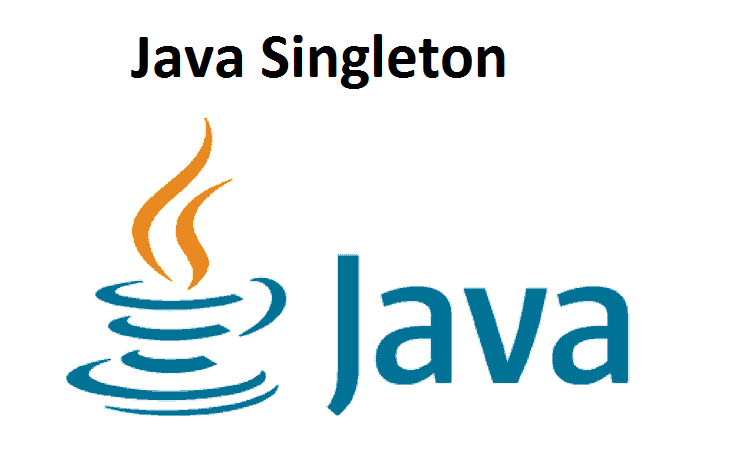#### Java Singleton

Java Singleton In this tutorial, we will find out about the singleton design pattern and how to apply it in ...#### Java Anonymous Class

Java Anonymous Class Java Anonymous Class: In this tutorial, you will find out about Anonymous classes in Java with the ...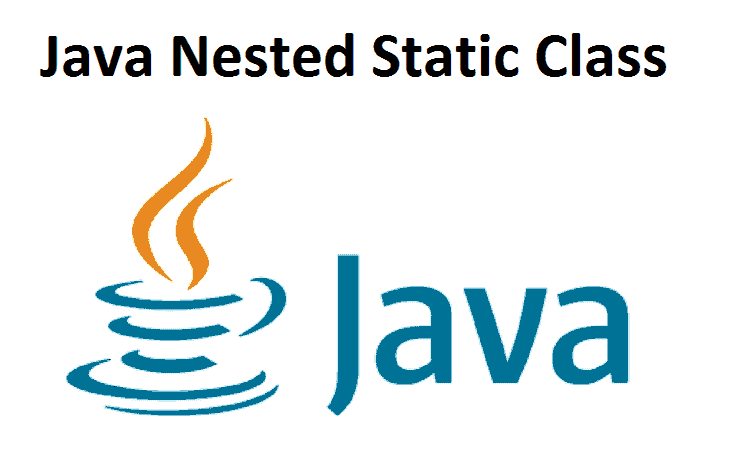#### Java Nested Static Class

Java Nested Static Class Java Nested Static Class: In this tutorial, you will find out about the nested static class ...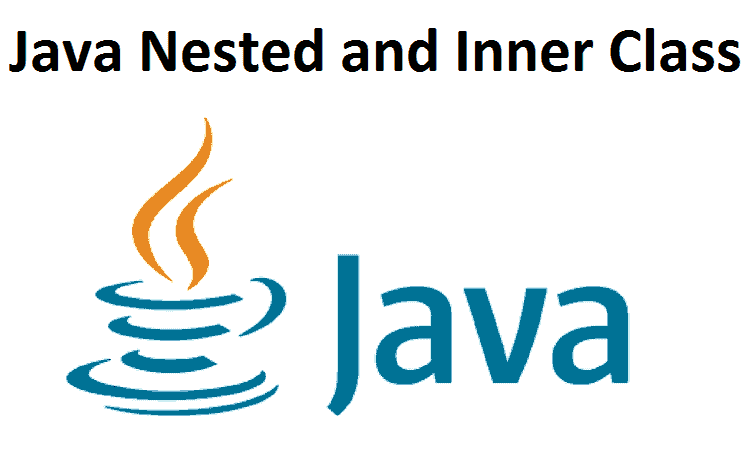#### Java Nested and Inner Class

Java Nested and Inner Class Java Nested and Inner Class: In this tutorial, you will find out about the nested ...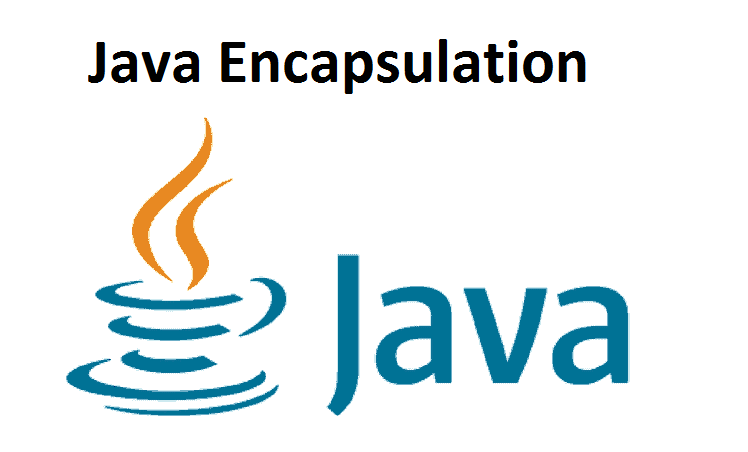#### Java Encapsulation

Java Encapsulation In this tutorial, you will find out about encapsulation and data hiding in Java with the help of ...#### Java Polymorphism

Java Polymorphism In this tutorial, we will find out about Java polymorphism and its implementation with the help of examples ...#### Java Interface

Java Interface Java Interface: In this tutorial, we will find out about Java interfaces. We will learn how to implement ...#### Java Abstract Class and Abstract Methods

Java Abstract Class and Abstract Methods In this tutorial, we will find out about Abstraction in Java. We will find ...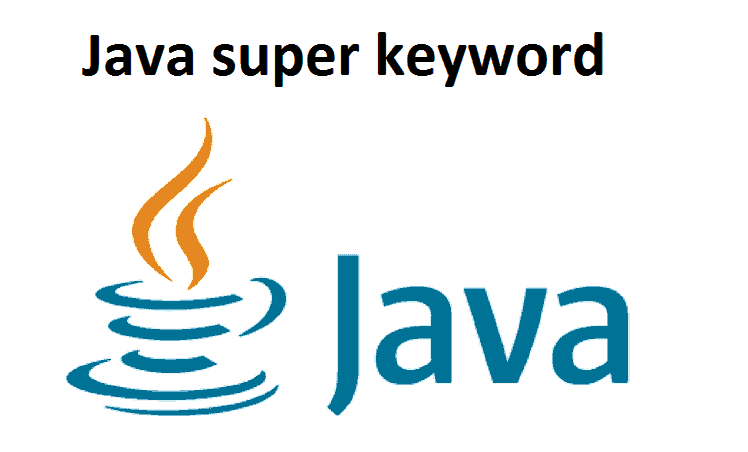#### Java super keyword

Java super keyword Java super keyword: In this tutorial, we will find out about the super keyword in Java with ...#### Java Method Overriding

Java Method Overriding In this tutorial, we will find out about the method overriding in Java with the help of ...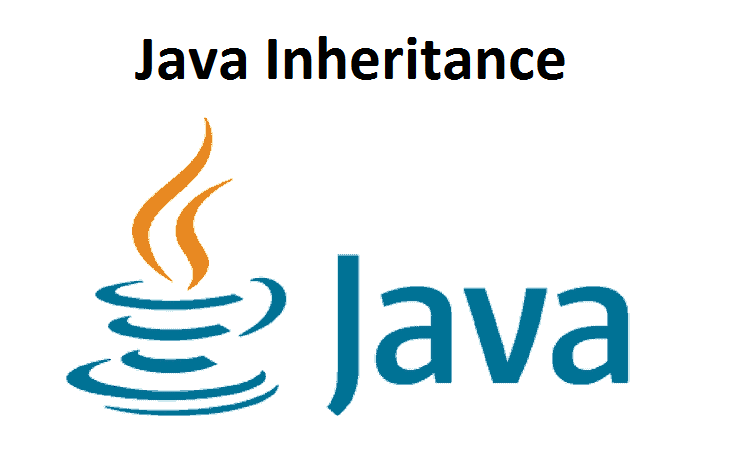#### Java Inheritance

Java Inheritance In this tutorial, we will find out about inheritance in Java with the help of examples. Inheritance is ...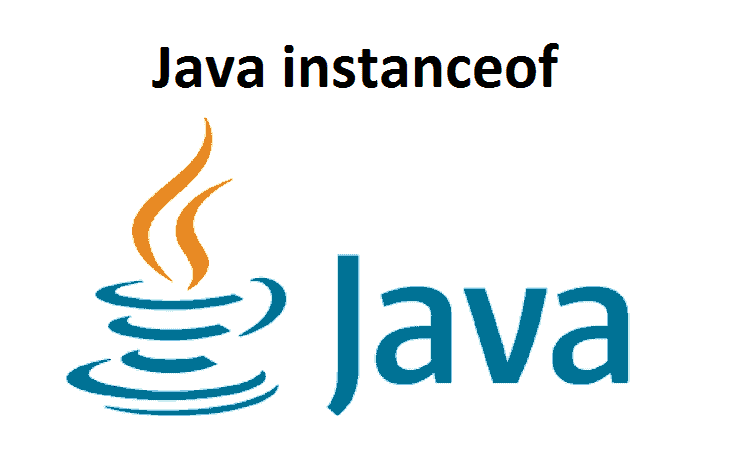#### Java instanceof

Java instanceof In this tutorial, you will find out about Java instanceof operator in detail with the help of examples ...#### Java Recursion

Java Recursion Java Recursion: In this tutorial, you will find out about Java recursive function, its advantages, and its disadvantages ...#### Java final keyword

Java final keyword Java final keyword: In this tutorial, we will find out about Java final variables, methods, and classes ...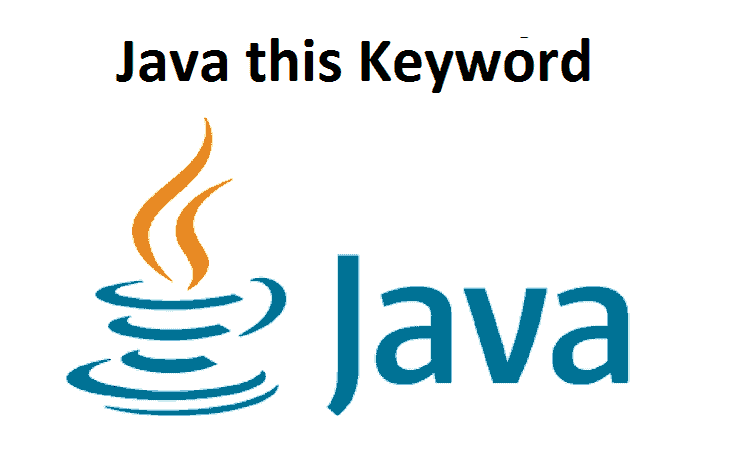#### Java this Keyword

Java this Keyword In this tutorial, we will find out about this keyword in Java, how and where to use ...#### Java Access Modifiers

Java Access Modifiers In this tutorial, we will find out about the Java Access Modifier, its sorts, and how to ...#### Java String

Java String In this tutorial, we will find out about Java String, how to make it, and its different methods ...#### Java Constructors

Java Constructors In this tutorial, you'll find out about Java constructors, how to make and use them, and various types ...